Like   Tweet   in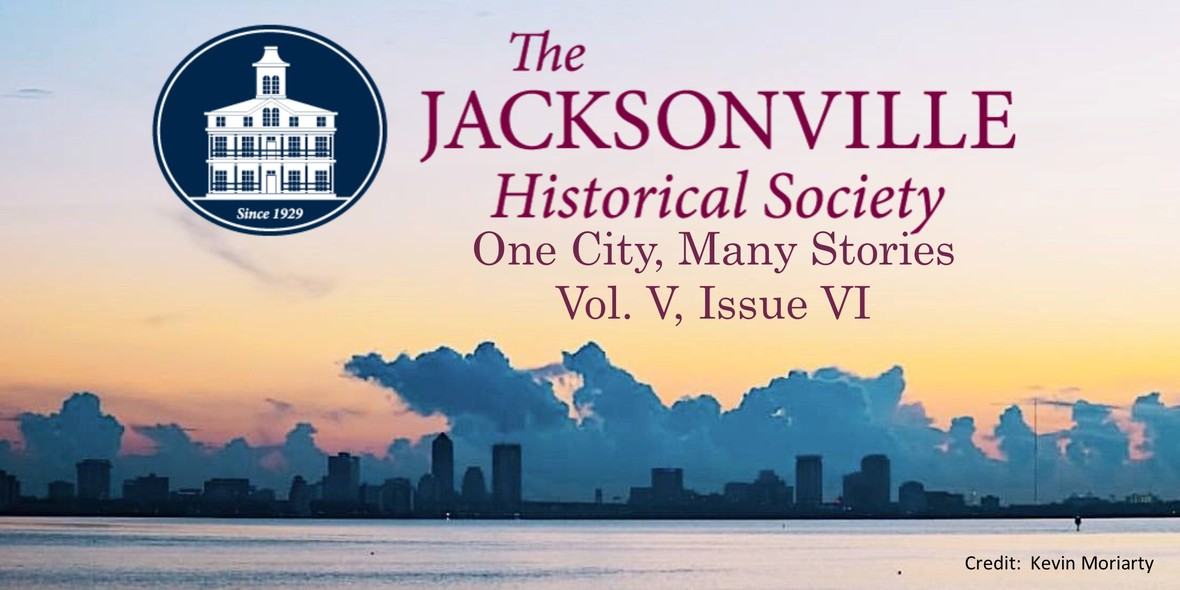# Jacksonville History Matters: JULY NEWS & EVENTS

 table div table+table+table+table div table{width:100%;padding:0}table div table+table+table+table div table img{width:96.23%;padding:0;float:none}table div table+table+table+table div table td{width:100%;padding:0 1.88% 18px}/* styles */## Speaking of Jacksonville History

 /* styles */ Readers of “Jacksonville History Matters” know that the Jacksonville Historical Society celebrated its 90th Anniversary last month. For the occasion, our staff and volunteers have been on the lookout for evidence of our own organizational history. An early list of speakers who have addressed the Society’s membership included some familiar, sometimes remarkable names.
 table div table+table+table+table+table+table+table div table,table.module-6{width:32.08%;float:left;padding:0}table div table+table+table+table+table+table+table div table a{border:0 none;text-decoration:none}table div table+table+table+table+table+table+table div table img{width:100%!important;border:0 none;text-decoration:none}table div table+table+table+table+table+table+table div table td{width:100%;padding:0 20px 20px 0}/* styles */ The earliest record of a program at the JHS is from May 12, 1948, when Dr. Frank Slaughter discussed the early history of Fernandina (as Fernandina Beach was then known). Dr. Slaughter (1908-2001) a highly-respected physician at Riverside Hospital and a genuine renaissance man. He was the prolific author of books both fiction and non-fiction, and had a strong interest in history. Over the ensuing decades Dr. Slaughter returned four times to the JHS, speaking on topics relating to Northeast Florida. The names of other early JHS guest speakers resonate among students of Florida history: Kathryn Abbey Hanna, Charlton Tebeau, Albert Manucy, Charles Arnade, Charles Fairbanks, Rembert Patrick and Jerrell Shofner – all Floridianists who produced significant scholarship. Dr. Hanna was the speaker on November 13, 1953, at a special meeting where the JHS hosted the Southern Historical Association. (Her topic title is unrecorded).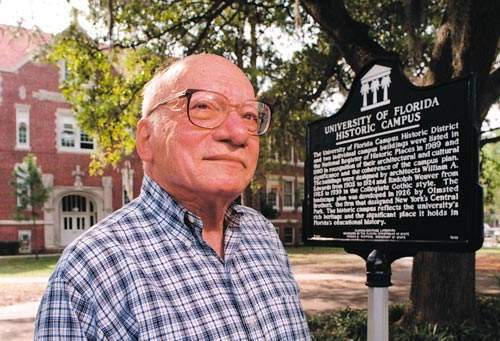Dr. Samuel Proctor, 1921 - 2005. Source: University of Florida
 /* styles */ On May 30, 1962 the topic was “Jacksonville During the Civil War.” The speaker was 44 year-old University of Florida historian Samuel Proctor. Thinking to study law, Sam Proctor (1919-2005), a Jacksonville native, arrived at the University of Florida in 1937. Diverted by an interest in history, he produced a 560-page master’s thesis about his fellow Jacksonvillian, Napoleon Bonaparte Broward, researched in the Browards’ home at 935 East Church Street. There Proctor worked among boxes and files that had been undisturbed since the late governor’s death in 1910. The result appeared in multiple editions, as Napolean Bonaparte Broward: Florida's Fighting Democrat (University Press of Florida, 1950), and the gracious Sam Proctor, with an encyclopedic command of Florida history, went on to train generations of students (including me) at his beloved University of Florida.
 table div table+table+table+table+table+table+table+table+table+table div table,table.module-9{width:32.08%;float:right;padding:0}table div table+table+table+table+table+table+table+table+table+table div table a{border:0 none;text-decoration:none}table div table+table+table+table+table+table+table+table+table+table div table img{width:100%!important;border:0 none;text-decoration:none}table div table+table+table+table+table+table+table+table+table+table div table td{width:100%;padding:0 0 20px 20px}/* styles */ On February 11, 1959, Mrs. Marjory Stoneman Douglas visited the JHS to speak about “Hurricanes and History.” Mrs. Douglas, by then 69 years of age, was widely-known as the author of The Everglades: River of Grass (Rinehart, 1947), part of the Rivers of American series. Her book went on to sell over half a million copies in numerous editions, and Mrs. Douglas lived to 108. She was a journalist, suffragist, author, staunch conservationist, life-long renaissance woman, and a legendary voice in Florida and environmental history.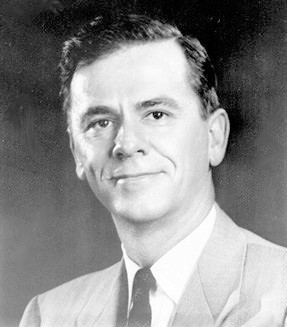Congressman Charles E. Bennett, 1910 - 2003

Elected officials periodically graced the podium at the JHS, including the redoubtable Charles E. Bennett (1910-2003), who represented Florida in the U.S. Congress from 1949 to 1993, making him the longest serving congressman in Florida’s history. On November 11, 1959, his topic was dear to his history-minded soul: “Future Plans for the Ft. Caroline Memorial.” That memorial exists today as part of the Timucuan Ecological and Historic Preserve, both of which owe heavily to the efforts of Congressman Bennett – himself an amateur historian who wrote and published numerous books and articles on the history of North Florida.

In the twenty-first century, Speaker Series programs at the Jacksonville Historical Society maintain their reputation for excellence while gaining in popularity. Since 1998 we have met monthly at Old St. Andrew’s, next to the Baseball Grounds and across the street from the entrance to the Vystar Veteran’s Memorial Arena. Strong attendance sometimes requires us to meet at a larger venue, as was the case with this May’s annual meeting. To ensure that you have priority to register for events, join (or renew your membership in) the JHS. Most events are free for members! Summer and Fall events are listed below.

Alan Bliss
Executive Director

 table div table+table+table+table+table+table+table+table+table+table+table+table+table div table{width:100%;padding:0}table div table+table+table+table+table+table+table+table+table+table+table+table+table div table img{width:96.23%;padding:0;float:none}table div table+table+table+table+table+table+table+table+table+table+table+table+table div table td{width:100%;padding:0 1.88% 18px}/* styles */## JULY 13: Salute to the Jax Red Caps & Game!

 table div table+table+table+table+table+table+table+table+table+table+table+table+table+table+table div table,table.module-14{width:32.08%;float:right;padding:0}table div table+table+table+table+table+table+table+table+table+table+table+table+table+table+table div table a{border:0 none;text-decoration:none}table div table+table+table+table+table+table+table+table+table+table+table+table+table+table+table div table img{width:100%!important;border:0 none;text-decoration:none}table div table+table+table+table+table+table+table+table+table+table+table+table+table+table+table div table td{width:100%;padding:0 0 20px 20px}/* styles */ Tickets available here. Enjoy an action-packed night with your JHS and Durkeeville friends while we immerse ourselves in the Negro League history and enjoy the Jumbo Shrimp Salute to the Jax Red Caps! 5:00pm Salute to the Jax Red Caps presented by the Durkeeville Historical Society and the Jacksonville Historical Society. 6:00pm Walk next door to the ballpark with JHS and Durkeeville friends. 6:30pm Enjoy the Jax Jumbo Shrimp "Salute to the Red Caps" game in Section 110 with friends from JHS and Durkeeville Historical Society! Seats are located behind home plate on first base line. Parking behind Old St. Andrews is included in your ticket price. GET YOUR TICKETS HERE!
 table div table+table+table+table+table+table+table+table+table+table+table+table+table+table+table+table+table div table{width:100%;padding:0}table div table+table+table+table+table+table+table+table+table+table+table+table+table+table+table+table+table div table img{width:96.23%;padding:0;float:none}table div table+table+table+table+table+table+table+table+table+table+table+table+table+table+table+table+table div table td{width:100%;padding:0 1.88% 18px}/* styles */## This just in...Jacksonville has many stories to tell

 /* styles */ The archives at the Jacksonville Historical Society are a vast wealth of knowledge for our researchers and visitors, but our library is nothing to sneeze at either. In addition to our city directories, high school yearbooks and myriad of books focusing on Jacksonville history, you can also find some more unusual items lurking on the shelves. One example is our set of Editorial Comment from our local CBS affiliate,WJXT.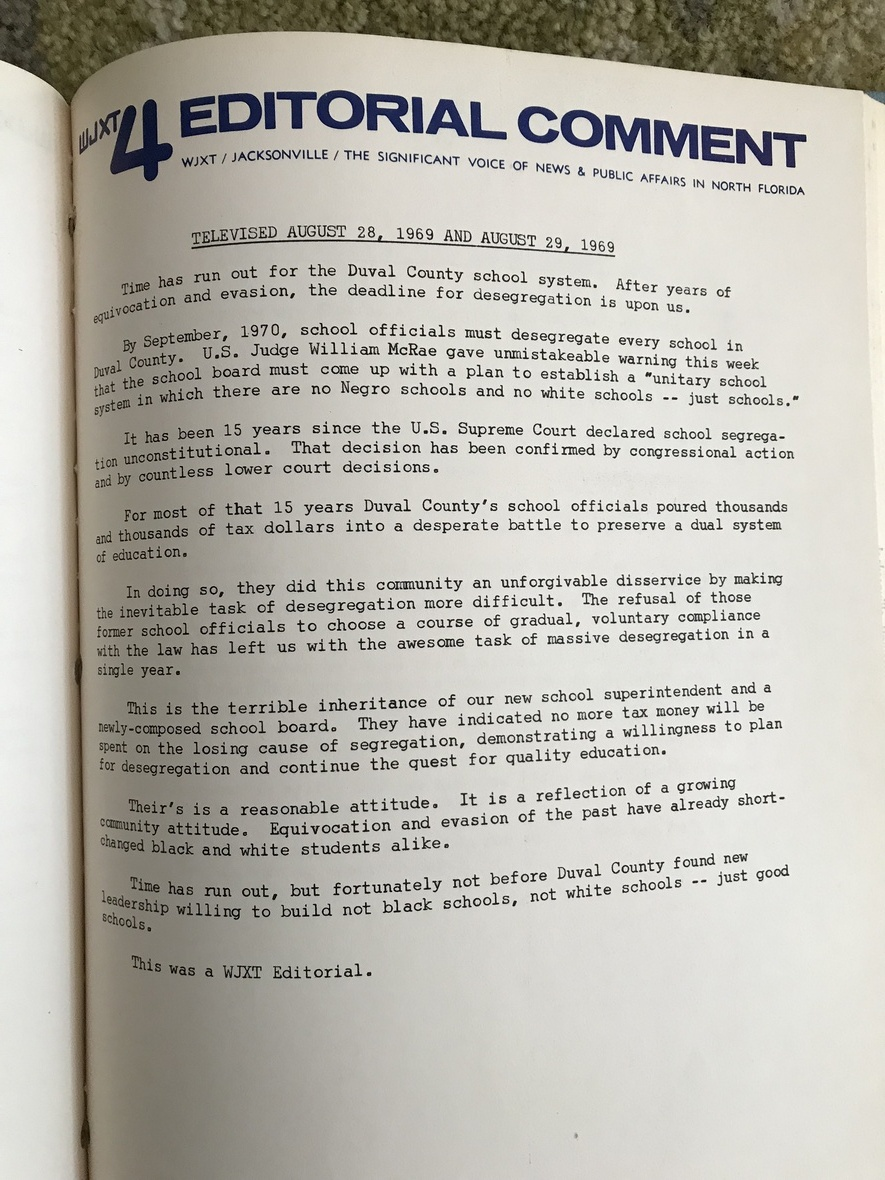Transcript of WJXT editorial comment (August 28, 1969)
 /* styles */ Spanning the 1960s and 1970s, each transcript contains a snapshot of the biggest news of the day along with the date it was televised. I pulled the volume from 1969 off of the shelf in hopes of seeing what the hot button issues were 50 years ago. As you can imagine, 1969 was a tumultuous year in American history, and that was certainly the case in Duval County. The wide variety of topics covered include how Mayor Tanzler is handling a recently consolidated government, a looming deadline to desegregate our public schools, and even a piece on taking a second look at the dangers of marijuana use. The more you flip through these volumes, the more you realize that this city has always been, and likely will always be, a whirlwind of debate. There is, however, one area in which I'm sure all of us would agree; this great big city has many stories to tell. We are committed to preserving those stories so that future generations can benefit from the them. by Mitch Hemann Archivist
 table div table+table+table+table+table+table+table+table+table+table+table+table+table+table+table+table+table+table+table+table+table+table div table{width:100%;padding:0}table div table+table+table+table+table+table+table+table+table+table+table+table+table+table+table+table+table+table+table+table+table+table div table img{width:96.23%;padding:0;float:none}table div table+table+table+table+table+table+table+table+table+table+table+table+table+table+table+table+table+table+table+table+table+table div table td{width:100%;padding:0 1.88% 18px}/* styles */table.module-23{width:75.47%;padding:0}table div table+table+table+table+table+table+table+table+table+table+table+table+table+table+table+table+table+table+table+table+table+table+table+table div table{width:75.47%;float:none;margin-left:auto;margin-right:auto;padding:0}table div table+table+table+table+table+table+table+table+table+table+table+table+table+table+table+table+table+table+table+table+table+table+table+table div table a{border:0 none;text-decoration:none}table div table+table+table+table+table+table+table+table+table+table+table+table+table+table+table+table+table+table+table+table+table+table+table+table div table img{width:100%!important;border:0 none;text-decoration:none}table div table+table+table+table+table+table+table+table+table+table+table+table+table+table+table+table+table+table+table+table+table+table+table+table div table td{width:100%;padding:0}/* styles */
 table div table+table+table+table+table+table+table+table+table+table+table+table+table+table+table+table+table+table+table+table+table+table+table+table+table+table+table div table{width:100%;padding:0}table div table+table+table+table+table+table+table+table+table+table+table+table+table+table+table+table+table+table+table+table+table+table+table+table+table+table+table div table img{width:96.23%;padding:0;float:none}table div table+table+table+table+table+table+table+table+table+table+table+table+table+table+table+table+table+table+table+table+table+table+table+table+table+table+table div table td{width:100%;padding:0 1.88% 18px}/* styles */table.module-27{width:75.47%;padding:0}table div table+table+table+table+table+table+table+table+table+table+table+table+table+table+table+table+table+table+table+table+table+table+table+table+table+table+table+table div table{width:75.47%;float:none;margin-left:auto;margin-right:auto;padding:0}table div table+table+table+table+table+table+table+table+table+table+table+table+table+table+table+table+table+table+table+table+table+table+table+table+table+table+table+table div table a{border:0 none;text-decoration:none}table div table+table+table+table+table+table+table+table+table+table+table+table+table+table+table+table+table+table+table+table+table+table+table+table+table+table+table+table div table img{width:100%!important;border:0 none;text-decoration:none}table div table+table+table+table+table+table+table+table+table+table+table+table+table+table+table+table+table+table+table+table+table+table+table+table+table+table+table+table div table td{width:100%;padding:0}/* styles */

## Gingerbread Builders' Workshop - September 14

 /* styles */ This is your chance. Get the advice we've all been waiting for ... Local experts and artists will instruct us on best practices for gingerbread construction on Saturday, September 14! This is a "DON'T MISS" event, if you are considering building for the 2019 Gingerbread Extravaganza. You will learn valuable tips and techniques in gingerbread construction and other edible building materials. This workshop will give you the combined confidence of Martha Stewart and Sir Isaac Newton and prepare you for a joyful building experience. Mark your calendar and reserve a spot.
 table div table+table+table+table+table+table+table+table+table+table+table+table+table+table+table+table+table+table+table+table+table+table+table+table+table+table+table+table+table+table+table+table div table{width:100%;padding:0}table div table+table+table+table+table+table+table+table+table+table+table+table+table+table+table+table+table+table+table+table+table+table+table+table+table+table+table+table+table+table+table+table div table img{width:96.23%;padding:0;float:none}table div table+table+table+table+table+table+table+table+table+table+table+table+table+table+table+table+table+table+table+table+table+table+table+table+table+table+table+table+table+table+table+table div table td{width:100%;padding:0 1.88% 18px}/* styles */table.module-32{width:75.47%;padding:0}table div table+table+table+table+table+table+table+table+table+table+table+table+table+table+table+table+table+table+table+table+table+table+table+table+table+table+table+table+table+table+table+table+table div table{width:75.47%;float:none;margin-left:auto;margin-right:auto;padding:0}table div table+table+table+table+table+table+table+table+table+table+table+table+table+table+table+table+table+table+table+table+table+table+table+table+table+table+table+table+table+table+table+table+table div table a{border:0 none;text-decoration:none}table div table+table+table+table+table+table+table+table+table+table+table+table+table+table+table+table+table+table+table+table+table+table+table+table+table+table+table+table+table+table+table+table+table div table img{width:100%!important;border:0 none;text-decoration:none}table div table+table+table+table+table+table+table+table+table+table+table+table+table+table+table+table+table+table+table+table+table+table+table+table+table+table+table+table+table+table+table+table+table div table td{width:100%;padding:0}/* styles */

## Holiday Party Reservations at Old St. Andrews

 /* styles */ Shake off the hassles and work of holiday party planning. Old St. Andrews and the Gingerbread Extravaganza are available for evening holiday parties this December! Impress your friends, clients or staff by hosting your holiday party in the ambiance of Old St. Andrews decorated in Gingerbread Extravaganza splendor. Our Rental Coordinators have catering resources ready to provide a turnkey event for you! For more information, contact our Rental Coordinators: Jade Stanley: 904-878-8122 Rob Jackson: 904-878-2241 Email: Events@JaxHistory.org
 table div table+table+table+table+table+table+table+table+table+table+table+table+table+table+table+table+table+table+table+table+table+table+table+table+table+table+table+table+table+table+table+table+table+table+table+table div table{width:100%;padding:0}table div table+table+table+table+table+table+table+table+table+table+table+table+table+table+table+table+table+table+table+table+table+table+table+table+table+table+table+table+table+table+table+table+table+table+table+table div table img{width:96.23%;padding:0;float:none}table div table+table+table+table+table+table+table+table+table+table+table+table+table+table+table+table+table+table+table+table+table+table+table+table+table+table+table+table+table+table+table+table+table+table+table+table div table td{width:100%;padding:0 1.88% 18px}/* styles */## J. F. Bryan IV joins JHS Board

 table div table+table+table+table+table+table+table+table+table+table+table+table+table+table+table+table+table+table+table+table+table+table+table+table+table+table+table+table+table+table+table+table+table+table+table+table+table+table div table,table.module-37{width:32.08%;float:right;padding:0}table div table+table+table+table+table+table+table+table+table+table+table+table+table+table+table+table+table+table+table+table+table+table+table+table+table+table+table+table+table+table+table+table+table+table+table+table+table+table div table a{border:0 none;text-decoration:none}table div table+table+table+table+table+table+table+table+table+table+table+table+table+table+table+table+table+table+table+table+table+table+table+table+table+table+table+table+table+table+table+table+table+table+table+table+table+table div table img{width:100%!important;border:0 none;text-decoration:none}table div table+table+table+table+table+table+table+table+table+table+table+table+table+table+table+table+table+table+table+table+table+table+table+table+table+table+table+table+table+table+table+table+table+table+table+table+table+table div table td{width:100%;padding:0 0 20px 20px}/* styles */ The Jacksonville Historical Society is pleased to announce that J.F. Bryan, IV has joined its Board of Directors. Mr. Bryan, a native of Jacksonville, was a leader in the insurance industry for thirty years. He was President of the Jacksonville-based Independent Insurance Group until 1996, when that company was sold to American General. Mr. Bryan now serves as a community trustee working with a variety of civic and charitable organizations. Mr. Bryan’s record of public service and stewardship is well-known, in and beyond Jacksonville. Currently he is Chairman of the Advisory Board of the Local Initiatives Support Corporation (LISC), one of the country's largest organizations supporting projects to revitalize communities and bring greater economic opportunity to residents. He is Chairman of the Paul E. and Klare N. Reinhold Foundation, Inc., and is a member of the Board of Jacksonville University (from which he received an Honorary Degree in 2011 for his twenty-four years of board service). He is also on the Board and Past Chairman of the Jacksonville Symphony Association, Chairman of Ronald McDonald House Advisory Board, Jacksonville Civic Council, the Schultz Center for Teaching & Leadership, the board of Evergreen Funeral Home and Crematory, Bok Tower Gardens in Lake Wales, FL, and of the James Madison Institute in Tallahassee. He is a Trustee of The Thomas Jefferson Foundation, stewards of Jefferson’s home, Monticello and of Jefferson’s ideas. In the past, Mr. Bryan has served as President or Chairman of the Board of the Arts Assembly, Big Brothers, The Community Foundation, Episcopal Children Services Foundation, the Jacksonville Zoo, Leadership Jacksonville, Meninak, the Jacksonville Symphony Association, Evergreen Cemetery, Episcopal High School Foundation Board and Bethune-Cookman College). He was a member of the board of Jacksonville Community Council, Inc. (JCCI) where he chaired two studies (City Finance and Public Education), the Non-Profit Center, Episcopal Relief and Development in New York, as well as serving a six-year term as Regent of the University of the South, his alma mater. Mr. Bryan holds a B.A. in history from the University of the South. He has been a life-long active member of the Episcopal Church of the Good Shepherd in Jacksonville. He and his wife, Peggy, are the parents of three sons and six grandchildren.
 table div table+table+table+table+table+table+table+table+table+table+table+table+table+table+table+table+table+table+table+table+table+table+table+table+table+table+table+table+table+table+table+table+table+table+table+table+table+table+table div table{width:100%;padding:0}table div table+table+table+table+table+table+table+table+table+table+table+table+table+table+table+table+table+table+table+table+table+table+table+table+table+table+table+table+table+table+table+table+table+table+table+table+table+table+table div table img{width:96.23%;padding:0;float:none}table div table+table+table+table+table+table+table+table+table+table+table+table+table+table+table+table+table+table+table+table+table+table+table+table+table+table+table+table+table+table+table+table+table+table+table+table+table+table+table div table td{width:100%;padding:0 1.88% 18px}/* styles */## Independence Day Hours

 table.module-40{width:75.47%;padding:0}table div table+table+table+table+table+table+table+table+table+table+table+table+table+table+table+table+table+table+table+table+table+table+table+table+table+table+table+table+table+table+table+table+table+table+table+table+table+table+table+table+table div table{width:75.47%;float:none;margin-left:auto;margin-right:auto;padding:0}table div table+table+table+table+table+table+table+table+table+table+table+table+table+table+table+table+table+table+table+table+table+table+table+table+table+table+table+table+table+table+table+table+table+table+table+table+table+table+table+table+table div table a{border:0 none;text-decoration:none}table div table+table+table+table+table+table+table+table+table+table+table+table+table+table+table+table+table+table+table+table+table+table+table+table+table+table+table+table+table+table+table+table+table+table+table+table+table+table+table+table+table div table img{width:100%!important;border:0 none;text-decoration:none}table div table+table+table+table+table+table+table+table+table+table+table+table+table+table+table+table+table+table+table+table+table+table+table+table+table+table+table+table+table+table+table+table+table+table+table+table+table+table+table+table+table div table td{width:100%;padding:0}/* styles */
 table div table+table+table+table+table+table+table+table+table+table+table+table+table+table+table+table+table+table+table+table+table+table+table+table+table+table+table+table+table+table+table+table+table+table+table+table+table+table+table+table+table+table div table{width:100%;padding:0}table div table+table+table+table+table+table+table+table+table+table+table+table+table+table+table+table+table+table+table+table+table+table+table+table+table+table+table+table+table+table+table+table+table+table+table+table+table+table+table+table+table+table div table img{width:96.23%;padding:0;float:none}table div table+table+table+table+table+table+table+table+table+table+table+table+table+table+table+table+table+table+table+table+table+table+table+table+table+table+table+table+table+table+table+table+table+table+table+table+table+table+table+table+table+table div table td{width:100%;padding:0 1.88% 18px}/* styles */## Intern Industry

 table.module-43{width:75.47%;padding:0}table div table+table+table+table+table+table+table+table+table+table+table+table+table+table+table+table+table+table+table+table+table+table+table+table+table+table+table+table+table+table+table+table+table+table+table+table+table+table+table+table+table+table+table+table div table{width:75.47%;float:none;margin-left:auto;margin-right:auto;padding:0}table div table+table+table+table+table+table+table+table+table+table+table+table+table+table+table+table+table+table+table+table+table+table+table+table+table+table+table+table+table+table+table+table+table+table+table+table+table+table+table+table+table+table+table+table div table a{border:0 none;text-decoration:none}table div table+table+table+table+table+table+table+table+table+table+table+table+table+table+table+table+table+table+table+table+table+table+table+table+table+table+table+table+table+table+table+table+table+table+table+table+table+table+table+table+table+table+table+table div table img{width:100%!important;border:0 none;text-decoration:none}table div table+table+table+table+table+table+table+table+table+table+table+table+table+table+table+table+table+table+table+table+table+table+table+table+table+table+table+table+table+table+table+table+table+table+table+table+table+table+table+table+table+table+table+table div table td{width:100%;padding:0}/* styles */
 /* styles */ Summer has brought an abundance of curiosity, youthfulness and energy to JHS. We are blessed to have the assistance of three high schools interns this summer. Each of these young people brought their own talents and spirit to the job. They have joined us to tackle many projects, including fundraiser planning, inventories, membership administration, archival work and Merrill House tours. We are grateful for their assistance and we wish them a fun and relaxing summer before they return to school!
 table div table+table+table+table+table+table+table+table+table+table+table+table+table+table+table+table+table+table+table+table+table+table+table+table+table+table+table+table+table+table+table+table+table+table+table+table+table+table+table+table+table+table+table+table+table+table div table{width:100%;padding:0}table div table+table+table+table+table+table+table+table+table+table+table+table+table+table+table+table+table+table+table+table+table+table+table+table+table+table+table+table+table+table+table+table+table+table+table+table+table+table+table+table+table+table+table+table+table+table div table img{width:96.23%;padding:0;float:none}table div table+table+table+table+table+table+table+table+table+table+table+table+table+table+table+table+table+table+table+table+table+table+table+table+table+table+table+table+table+table+table+table+table+table+table+table+table+table+table+table+table+table+table+table+table+table div table td{width:100%;padding:0 1.88% 18px}/* styles */## Summer and Fall Events

 table.module-47{width:75.47%;padding:0}table div table+table+table+table+table+table+table+table+table+table+table+table+table+table+table+table+table+table+table+table+table+table+table+table+table+table+table+table+table+table+table+table+table+table+table+table+table+table+table+table+table+table+table+table+table+table+table+table div table{width:75.47%;float:none;margin-left:auto;margin-right:auto;padding:0}table div table+table+table+table+table+table+table+table+table+table+table+table+table+table+table+table+table+table+table+table+table+table+table+table+table+table+table+table+table+table+table+table+table+table+table+table+table+table+table+table+table+table+table+table+table+table+table+table div table a{border:0 none;text-decoration:none}table div table+table+table+table+table+table+table+table+table+table+table+table+table+table+table+table+table+table+table+table+table+table+table+table+table+table+table+table+table+table+table+table+table+table+table+table+table+table+table+table+table+table+table+table+table+table+table+table div table img{width:100%!important;border:0 none;text-decoration:none}table div table+table+table+table+table+table+table+table+table+table+table+table+table+table+table+table+table+table+table+table+table+table+table+table+table+table+table+table+table+table+table+table+table+table+table+table+table+table+table+table+table+table+table+table+table+table+table+table div table td{width:100%;padding:0}/* styles */

## Deep Roots, Many Branches - The Musical Journey

 table.module-49{width:75.66%;padding:0}table div table+table+table+table+table+table+table+table+table+table+table+table+table+table+table+table+table+table+table+table+table+table+table+table+table+table+table+table+table+table+table+table+table+table+table+table+table+table+table+table+table+table+table+table+table+table+table+table+table+table div table{width:75.66%;float:none;margin-left:auto;margin-right:auto;padding:0}table div table+table+table+table+table+table+table+table+table+table+table+table+table+table+table+table+table+table+table+table+table+table+table+table+table+table+table+table+table+table+table+table+table+table+table+table+table+table+table+table+table+table+table+table+table+table+table+table+table+table div table a{border:0 none;text-decoration:none}table div table+table+table+table+table+table+table+table+table+table+table+table+table+table+table+table+table+table+table+table+table+table+table+table+table+table+table+table+table+table+table+table+table+table+table+table+table+table+table+table+table+table+table+table+table+table+table+table+table+table div table img{width:100%!important;border:0 none;text-decoration:none}table div table+table+table+table+table+table+table+table+table+table+table+table+table+table+table+table+table+table+table+table+table+table+table+table+table+table+table+table+table+table+table+table+table+table+table+table+table+table+table+table+table+table+table+table+table+table+table+table+table+table div table td{width:100%;padding:0}/* styles */
 /* styles */ Thank you to all the music lovers who came out to enjoy a fascinating journey through Northeast Florida Blues history on Thursday, June 27! Mitch Hemann, JHS Archivist, took us deep into the socio-economic origins of blues in the late 19th and 20th centuries while sharing stories of the major influencers of this musical genre in their societal context. It was a great night for connecting with fellow music lovers and local blues talent. Thank you, Mitch, for your passionate research of this rich legacy! JHS is blessed to have this talented young man on its staff.
 table div table+table+table+table+table+table+table+table+table+table+table+table+table+table+table+table+table+table+table+table+table+table+table+table+table+table+table+table+table+table+table+table+table+table+table+table+table+table+table+table+table+table+table+table+table+table+table+table+table+table+table+table div table{width:100%;padding:0}table div table+table+table+table+table+table+table+table+table+table+table+table+table+table+table+table+table+table+table+table+table+table+table+table+table+table+table+table+table+table+table+table+table+table+table+table+table+table+table+table+table+table+table+table+table+table+table+table+table+table+table+table div table img{width:96.23%;padding:0;float:none}table div table+table+table+table+table+table+table+table+table+table+table+table+table+table+table+table+table+table+table+table+table+table+table+table+table+table+table+table+table+table+table+table+table+table+table+table+table+table+table+table+table+table+table+table+table+table+table+table+table+table+table+table div table td{width:100%;padding:0 1.88% 18px}/* styles */## Our Mission:

To educate and inspire the greater Jacksonville community to value its history, by fostering understanding of how the region's past shapes our present.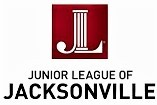## Thank you for your support!

 table div table+table+table+table+table+table+table+table+table+table+table+table+table+table+table+table+table+table+table+table+table+table+table+table+table+table+table+table+table+table+table+table+table+table+table+table+table+table+table+table+table+table+table+table+table+table+table+table+table+table+table+table+table+table+table+table+table div table{width:100%;padding:0}table div table+table+table+table+table+table+table+table+table+table+table+table+table+table+table+table+table+table+table+table+table+table+table+table+table+table+table+table+table+table+table+table+table+table+table+table+table+table+table+table+table+table+table+table+table+table+table+table+table+table+table+table+table+table+table+table+table div table img{width:96.23%;padding:0;float:none}table div table+table+table+table+table+table+table+table+table+table+table+table+table+table+table+table+table+table+table+table+table+table+table+table+table+table+table+table+table+table+table+table+table+table+table+table+table+table+table+table+table+table+table+table+table+table+table+table+table+table+table+table+table+table+table+table+table div table td{width:100%;padding:0 1.88% 18px}/* styles */## Staff:

Alan Bliss, Ph.D. , Executive Director | Mitch Hemann, Archivist | Susan Prattos, Administrator | Imani Phillips, Archives & Office Assistant | Sherrard Ceglia, Archives Assistant | Anna Verney, Archives Assistant | David Woodard, Facilities Manager